International
Tables for
Crystallography
Volume D
Physical properties of crystals
Edited by A. Authier

International Tables for Crystallography (2006). Vol. D, ch. 1.9, pp. 228-232

## Section 1.9.2. The atomic displacement parameters (ADPs)

W. F. Kuhsa*

aGZG Abt. Kristallographie, Goldschmidtstrasse 1, 37077 Göttingen, Germany
Correspondence e-mail: wkuhs1@gwdg.de

### 1.9.2. The atomic displacement parameters (ADPs)

| top | pdf |

One notes that in the Gaussian approximation, the mean-square atomic displacements (composed of thermal and static contributions) are fully described by six coefficients βij, which transform on a change of the direct-lattice base (according to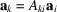) as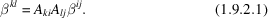This is the transformation law of a tensor (see Section 1.1.3.2); the mean-square atomic displacements are thus tensorial properties of an atom α. As the tensor is contravariant and in general is described in a (non-Cartesian) crystallographic basis system, its indices are written as superscripts. It is convenient for comparison purposes to quote the dimensionless coefficients βij as their dimensioned representations Uij.

In the harmonic approximation, the atomic displacements are fully described by the fully symmetric second-order tensor given in (1.9.2.1). Anharmonicity and disorder, however, cause deviations from a Gaussian distribution of the atomic displacements around the atomic position. In fact, anharmonicity in the thermal motion also provokes a shift of the atomic position as a function of temperature. A generalized description of atomic displacements therefore also involves first-, third-, fourth- and even higher-order displacement terms. These terms are defined by a moment-generating function M(Q) which expresses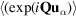in terms of an infinite number of moments; for a Gaussian distribution of displacement vectors, all moments of order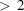are identically equal to zero. Thus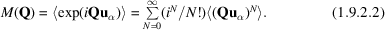The moments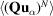of order N may be expressed in terms of cumulants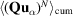by the identity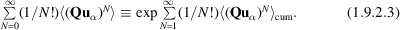Separating the powers of Q and u in (1.9.2.2)and (1.9.2.3), one may obtain expressions involving moments μ and cumulants k explicitly as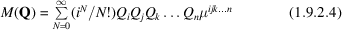and the cumulant-generating function K(Q) as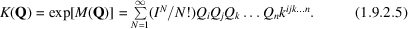The indices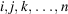run in three-dimensional space from 1 to 3 and refer to the crystallographic basis system. Moments may be expressed in terms of cumulants (and vice versa); the transformation laws are given in IT B (2001), equation (1.2.12.9) and more completely in Kuhs (1988, 1992). The moment- and cumulant-generating functions are two ways of expressing the Fourier transform of the atomic probability density function (p.d.f.). If all terms up to infinity are taken into account, M(Q) and K(Q) are [by virtue of the identity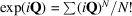] identical. For a finite series, however, the cumulants of order N carry implicit information on contributions of order N2, N3 etc. in contrast to the moments. Equations (1.9.2.4)and (1.9.2.5)are useful, as they can be entered directly in a structure-factor equation (see Chapter 1.2in IT B); however, the moments (and thus the cumulants) may also be calculated directly from the atomic p.d.f. as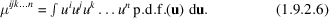The real-space expression of the p.d.f. obtained from a Fourier transform of (1.9.2.5)is called an Edgeworth series expansion. If one assumes that the underlying atomic p.d.f. is close to a Gaussian distribution, one may separate out the Gaussian contributions to the moment-generating function as suggested by Kuznetsov et al. (1960) and formulate a generating function for quasimoments as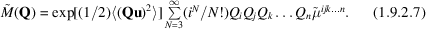These quasimoments are especially useful in crystallographic structure-factor equations, as they just modify the harmonic case. The real-space expression of the p.d.f. obtained from a Fourier transformation of (1.9.2.7)is called a Gram–Charlier series expansion. Discussions of its merits as compared to the Edgeworth series are given in Zucker & Schulz (1982a,b), Kuhs (1983, 1988, 1992) and Scheringer (1985).

#### 1.9.2.1. Tensorial properties of (quasi)moments and cumulants

| top | pdf |

By separating the powers of Q and u, one obtains in equations (1.9.2.4), (1.9.2.5)and (1.9.2.7)the higher-order displacement tensors in the form of moments, cumulants or quasimoments, which we shall denote in a general way as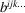; note that bij is identical to βij. They transform on a change of the direct-lattice base according to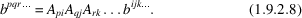The higher-order displacement tensors are fully symmetric with respect to the interchange of any of their indices; in the nomenclature of Jahn (1949), their tensor symmetry thus is [bN]. The number of independent tensor coefficients depends on the site symmetry of the atom and is tabulated in Sirotin (1960) as well as in Tables 1.9.3.1–1.9.3.6. For triclinic site symmetry, the numbers of independent tensor coefficients are 1, 3, 6, 10, 15, 21 and 28 for the zeroth to sixth order. Symmetry may further reduce the number of independent coefficients, as discussed in Section 1.9.3.

In many least-squares programs for structure refinement, the atomic displacement parameters are used in a dimensionless form [as given in (1.9.1.4)for the harmonic case]. These dimensionless quantities may be transformed according to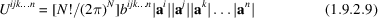(no summation) into quantities of units ÅN (or pmN); ai etc. are reciprocal-lattice vectors. Nowadays, the published structural results usually quote Uij for the second-order terms; it would be good practice to publish only dimensioned atomic displacements for the higher-order terms as well.

#### 1.9.2.2. Contraction, expansion and invariants of atomic displacement tensors

| top | pdf |

Anisotropic or higher-order atomic displacement tensors may contain a wealth of information. However, this information content is not always worth publishing in full, either because the physical meaning is not of importance or the significance is only marginal. Quantities of higher significance or better clarity are obtained by an operation known as tensor contraction. Likewise, lower-order terms may be expanded to higher order to impose certain (chemically implied) symmetries on the displacement tensors or to provide initial parameters for least-squares refinements. A contraction is obtained by multiplying the contravariant tensor components (referring to the real-space basis vectors) with the covariant components of the real-space metric tensor gij; for further details on tensor contraction, see Section 1.1.3.3.3. In the general case of atomic displacement tensors of (even) rank N, one obtains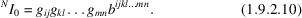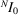is called the trace of a tensor of rank N and is a scalar invariant; it is given in units of lengthN and provides an easily interpretable quantity: In the case of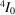, a positive sign indicates that the corresponding (real-space) p.d.f. is peaked, a negative sign indicates flatness of the p.d.f. The larger, the stronger the deviation from a Gaussian p.d.f. provoked by the atomic displacements of order N. The frequently quoted isotropic equivalent U value Ueq is also obtained by this contraction process. Noting that Uij may be expressed in terms of bij (= βij) according to (1.9.2.9)and that the trace of the matrix U is given as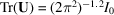, one obtains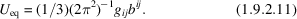Note that in all non-orthogonal bases,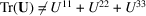. In older literature, the isotropic equivalent displacement parameter is often quoted as Beq, which is related to Ueq through the identity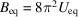. The use of Beq is now discouraged (Trueblood et al., 1996). Higher atomic displacement tensors of odd rank N may be reduced to simple vectors v by the following contraction: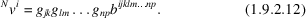where v1 is the 23 trace etc. Nvi is sometimes called a vector invariant, as it can be uniquely assigned to the tensor in question (Pach & Frey, 1964) and its units are lengthN − 1. The vector v is oriented along the line of maximum projected asymmetry for a given atom and vanishes for atoms with positional parameters fixed by symmetry; Johnson (1970) has named a vector closely related to 3v the vector of skew divergence. The calculation of v is useful as it gives the direction of the largest antisymmetric displacements contained in odd-rank higher-order thermal-motion tensors.

Atomic displacement tensors may also be partially contracted or expanded; rules for these operations are found in Kuhs (1992).

### References

International Tables for Crystallography (2001). Vol. B. Reciprocal space, edited by U. Shmueli. Dordrecht: Kluwer Academic Publishers.
Jahn, H. A. (1949). Note on the Bhagavantam–Suryanarayana method of enumerating the physical constants of crystals. Acta Cryst. 2, 30–33.
Johnson, C. K. (1970). Generalized treatments for thermal motion. In Thermal neutron diffraction, edited by B. T. M. Willis, pp. 132–160. Oxford University Press.
Kuhs, W. F. (1983). Statistical description of multimodal atomic probability densities. Acta Cryst. A39, 148–158.
Kuhs, W. F. (1988). The anharmonic temperature factor in crystallographic structure analysis. Aust. J. Phys. 41, 369–382.
Kuhs, W. F. (1992) Generalized atomic displacements in crystallographic structure analysis. Acta Cryst. A48, 80–98.
Kuznetsov, P. I., Stratonovich, R. L. & Tikhonov, V. I. (1960). Quasi-moment functions in the theory of random processes. Theory Probab. Its Appl. (USSR), 5, 80–97.
Pach, K. & Frey, T. (1964). Vector and tensor analysis. Budapest: Terra.
Scheringer, C. (1985). A deficiency of the cumulant expansion of the anharmonic temperature factor. Acta Cryst. A41, 79–81.
Sirotin, Yu. I. (1960). Group tensor spaces. Sov. Phys. Crystallogr. 5, 157–165.
Trueblood, K. N., Bürgi, H.-B., Burzlaff, H., Dunitz, J. D., Gramaccioli, C. M., Schulz, H. H., Shmueli, U. & Abrahams, S. C. (1996). Atomic displacement parameter nomenclature. Report of a subcommittee on atomic displacement parameter nomenclature. Acta Cryst. A52, 770–781.
Zucker, U. H. & Schulz, H. (1982a). Statistical approaches for the treatment of anharmonic thermal motion in crystals. I. A comparison of the most frequently used formalisms of anharmonic thermal vibrations. Acta Cryst. A38, 563–568.
Zucker, U. H. & Schulz, H. (1982b). Statistical approaches for the treatment of anharmonic thermal motion in crystals. II. Anharmonic thermal vibrations and effective atomic potentials in the fast ionic conductor lithium nitride Li3N. Acta Cryst. A38, 568–576.